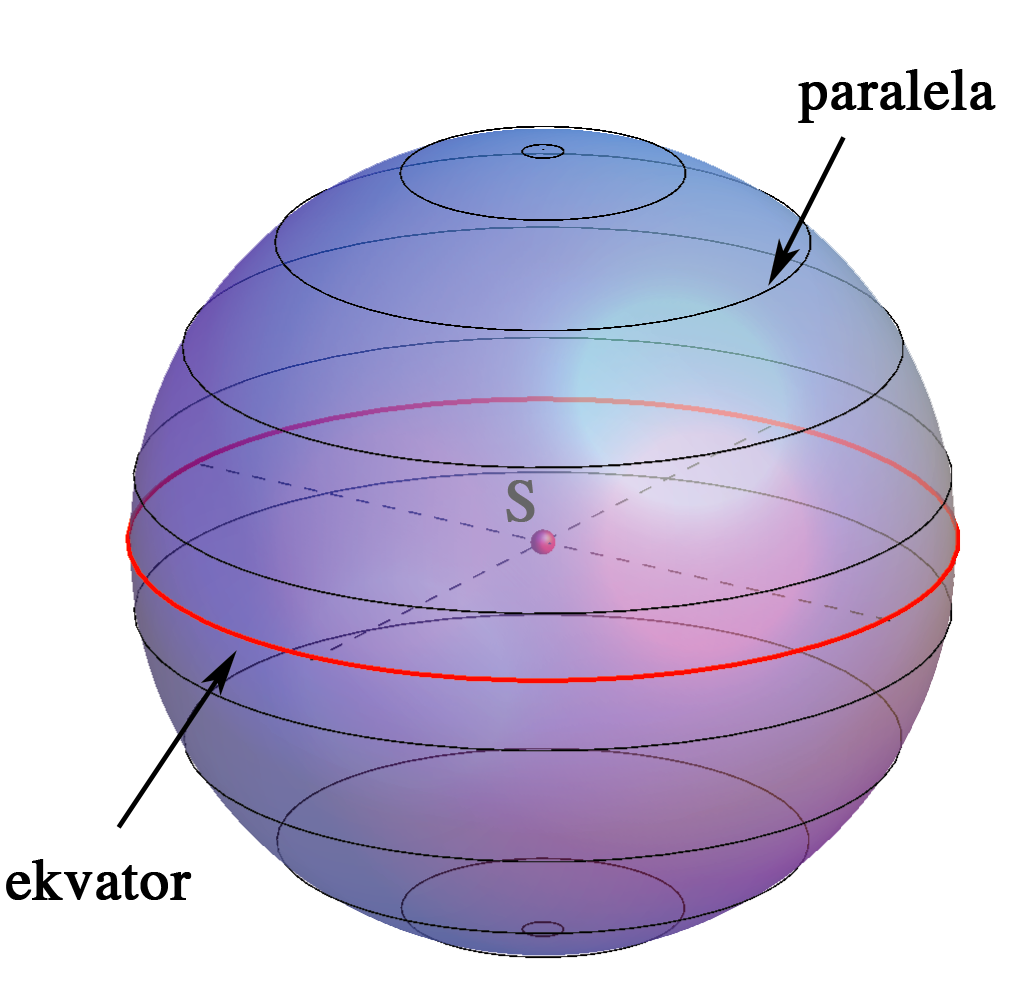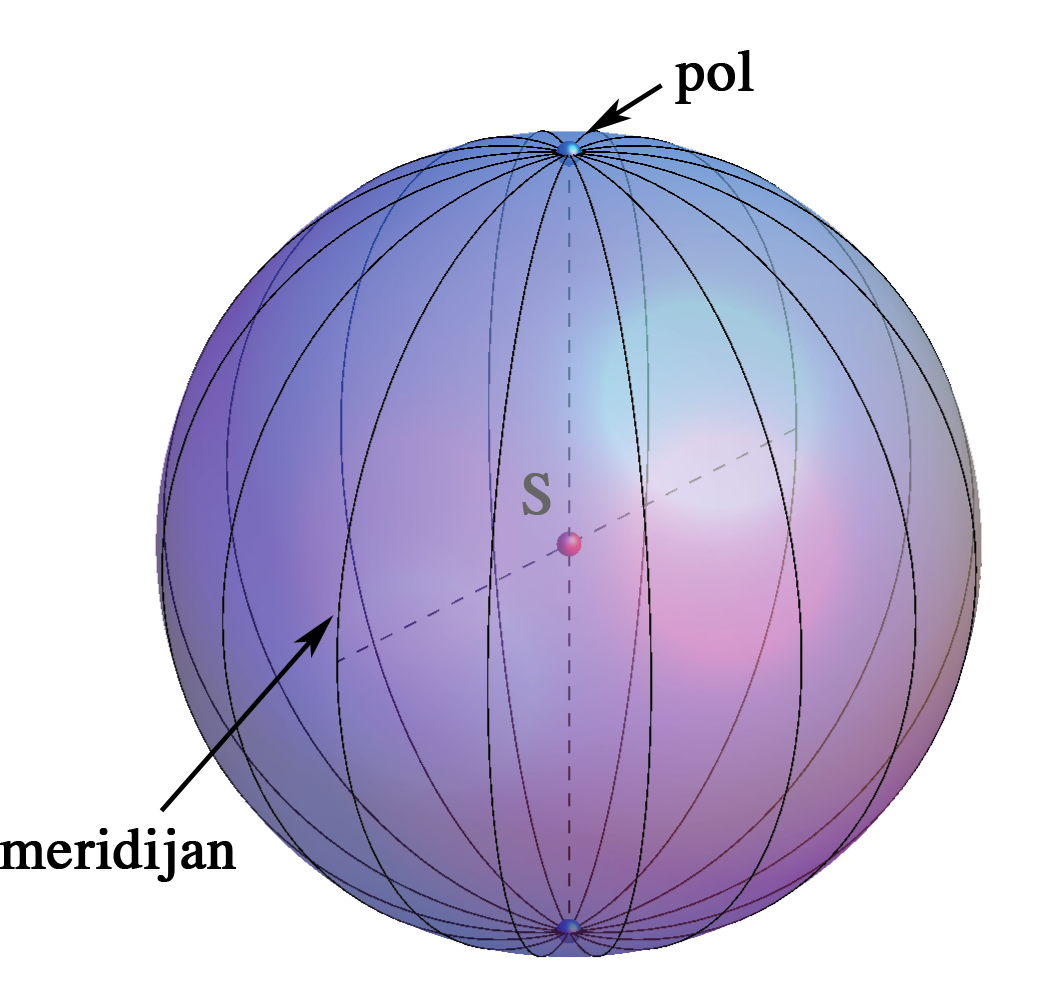## Sphere

• A sphere is a locus of points in the space that are at distance r from a fixed point S.

The point S is called a center of the sphere, the positive number r is called a radius of the sphere.

• A plane intersection of a sphere is a circle.

A center of a plane intersection of a sphere is an orthogonal projection of the center of the sphere to the intersecting plane.

• If a plane contains the center of a sphere, this plane intersection is a circle called a great circle.

A radius of a great circle equals the radius of the sphere.

• All points of the sphere are regular points.

• A tangent plane of the sphere at the point T is a plane through T perpendicular to the line ST, i.e. the line ST is a normal line of the sphere at T.

 circle intersections a tangent plane great circles

right-click to start the animation

• Intersections of horizontal planes and the sphere are called the lines of latitude, the longest among them is called an equator.

• Great circles that contain the endpoints of the vertical diameter of the sphere (poles) are called the lines of longitude or meridians.lines of latitude meridians-lines of longitude

#### Constructive assignments

• An intersection of a sphere and a line.
• A tangent plane of a sphere.
• An intersection of a sphere with a projecting plane.

Created by Sonja Gorjanc, translated by Helena Halas and Iva Kodrnja - 3DGeomTeh - Developing project of the University of Zagreb